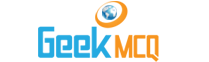# Engineering :: Thermodynamics

1.  1 atmospheric pressure is equal to
 A. 1 kg/cm2 B. 1.033 kg/cm2 C. 1.1 kg/cm2 D. 1.133 kg/cm2 E. 14.7 kg/cm2

2.  The general gas equation is
 A. PV = RT B. PV = mRT C. PV = (RT)m D. PVn = constant E. PV = constant

3.  The slope of constant pressure line on temperature entropy diagrm is given by
 A. S/T B. T/S C. Cp/T D. T/Cp E. 90?

4.  For water, boiling and melting point will concide at
 A. 0? K B. 100? K C. 200? K D. 2730.16? K E. 216.73? K

5.  On a Mollier chart the flow through turbine is represented by
 A. Horizontal line B. Vertical line C. Curved line convex up D. Carved line convex down E. None of the above

6.  In a steam turbine when steam expands, the entropy
 A. Increases linearly B. Decreases linearly C. Increases exponentially D. Decreases exponentially E. Does not change

7.  An isolated system
 A. Is a specified regon where transfers of energy and/or mass takes place B. Is a region of constant mass and only energy is allowed to cross the boundaries C. Cannot transfer either energy or mass to or from the surroundings D. Is one in which mass within the system is not necessarily constant E. None of the above

8.  The fact that the product of pressure and volume of a fixed amount of gas is of approximately constant value is known as
 A. Charle's law B. Gay Lussac's law C. Dalton's law D. Lewis law E. None of these

9.  The statement that energy can be neigher created nor destroyed but only converted from one form to another, is known as
 A. Kinetic theory of gases B. Avogadro's hypothesis C. Zerothlaw of thermodynamics D. First law of theormodynamics E. Second law of thermodynamics

10.  The sequence of processes that eventually returns the working substance to its original state is known as
 A. Event B. Process C. Thermodynamic property D. Thermodynamic cycle E. Zeroth law of thermodynamics